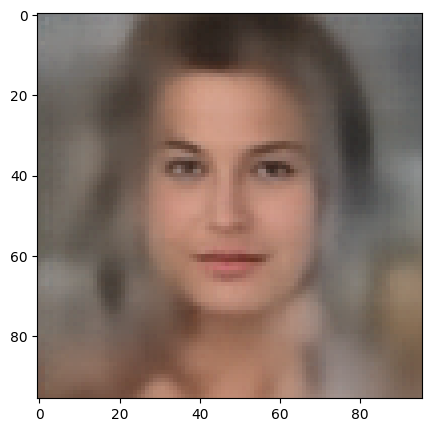# Latent spaces – pitfalls of distributing points in multi dimensions – I – constant probability density per dimension

This post requires Javascript to display formulas!

Sometimes when we solve some problems with Deep Neural Networks we have no direct clue about how such a network evolves during training and how exactly it encodes knowledge learned from patterns in the input data. The encoding may not only affect weights of neurons in neural filters or maps, but also the functional mapping of input data onto vectors of some intermediate or target vector space. To get a better understanding it may sometimes seem that experiments with statistical points or vectors in such vector spaces could be helpful. One could e.g. suggest to study the reaction of a trained Artificial Neural Network to input vectors distributed over a multidimensional feature space.

Another important case is the test of an Autoencoder’s Decoder network on data points which we distribute statistically over extended regions of the Autoencoder’s latent space.

An Autoencoder [AEs] is an example of a Encoder-Decoder pair of networks. The Encoder encodes original complex input data into vectors of the so called latent space – a multi-dimensional vector space. The Decoder instead uses vectors of the latent space to reconstruct or generate output data in the feature space of the original input objects. The dimensionality of the latent space may be much smaller than that of the original feature space, but still much bigger than the 2 or 3 dimensions we have intuitive experience with.

Latent spaces play a major role in Machine Learning [ML] whenever a deep neural algorithm encodes information. Embedding input data (like words or images) in a vector space by some special neural layers (e.g. for text or image classification) is nothing else than filling a kind of latent space with encoded data in an optimized way to help the neural network to solve a special task like e.g. text or image classification. But we can also use statistical data in the latent space of an AE together with its trained Decoder to create or better generate new objects. This leads us into the field of using neural networks for generative, creative tasks.

Unfortunately, we know from experience that not all data regions in a latent space support the production of objects which we would consider as being similar to our training objects or categories of such objects. A priori we actually know very little about the shape of the data distribution an encoding network will create during training. Therefore we may be tempted to scan the latent space in some way – and to distribute data points in it in some statistical way. And test a Decoder’s reaction to such points …in the hope to find an interesting region by chance.

In this small series of posts I want to point out that such statistical experiments may be futile. On the one hand side trying to fill a multidimensional space with “statistical” data may lead to unexpected and counter-intuitive results. On the other hand side you may easily miss interesting regions of such a vector space. Especially confined regions. The whole point is just another interesting variant of the curse of high dimensions.

The theoretical and numerical results which we will derive during the next posts underline the necessity to analyze the concrete data distributions a neural network creates in a latent space very carefully. Both regarding position and extension of the filled region – and maybe also with respect to sub-structures. The results will help us to understand better why an Autoencoder most often fails to produce reasonable objects from data points statistically distributed over larger regions of its latent space.

This post series requires an understanding of some basic math. In the first post I will derive some formulas describing data distributions resulting from a constant probability density for each vector component, i.e. a constant probability density per dimension. We will learn that with a growing number of dimensions the data points concentrate more and more in regions which are parts of a multi-dimensional sphere shell. Which is at least in my opinion a bit counter-intuitive and therefore an interesting finding in itself.

# Many dimensions …

An AE compresses data very effectively. So the dimension of a latent space will most often be significantly smaller than the number of dimensions M characterizing the feature space of the original training objects. The space spanned by logically independent variables which describe digitized objects may have a number of dimensions in the range of millions and tenths of millions. In the case of latent spaces we instead have to deal with a number of dimensions N in the range of multiples of 10 up to tens of thousands. (For relatively simple objects showing many correlations in their feature space …)

10 ≤ N ≤ 105    << M

Still, such numbers N are far beyond standard experience.

# Representation of a N-dimensional space by the ℜN

We can in general represent a multidimensional feature or latent space in Machine Learning by the ℜN. Each point in this space can be defined by a vector whose components correspond to the points’ coordinate values along the N orthogonal axes. A vector is thus represented by a N-tuple of real (coordinate) values:

(x1, x2, …, xk, … xN) .

Formally, the set of all tuples together with some addition and multiplication operations form a vector space over ℜ. Whether vector operations performed with vectors to different data points in a latent space or a feature space really have a solid interpretable meaning in your ML- or AE-context is not our business in this post. Our objective is to cover such a space with statistically defined points (or vectors).

# The curse of high dimensionality

It seems to be easy to fill a N-dimensional cube with data points that form a grid. More precisely, a grid with a constant distance between the points in all orthogonal directions parallel to the axes. However, a simple calculation shows that such an approach is doomed in computational practice:

Let us assume that we deal with a space of N = 256 dimensions. Let us also assume that we have reasons to believe that we should focus on the region of a cube around the origin that has a side length of 10 in each dimension k. Then the coordinate values xk for data points within the cube fulfill

$-b \lt x_{k} \lt +b \mbox{,} \quad \forall k \: \in [1,256] \quad \mbox{and} \quad b=5$

Let us further assume that we want to have a grid resolution of 10 points per dimension. Then we would need to create

$10^{256} \: \mbox{points}$

to fill our 256-dimensional cube with a homogeneous (!) point distribution. This is already a very clear indication that we can not systematically study a multidimensional cube in this way. Multidimensional spaces are huge by their nature.

We have to get and use more information about interesting regions or we must make assumptions on the distribution of points in each coordinate direction.

# Constant probability density per coordinate value and dimension

A most simple case for making an assumption is a constant probability distribution of each coordinate value xk within a defined interval along the related coordinate axis. Let us again pick a cube around the origin of a space with N=256:

$-b \lt x_{k} \lt +b, \quad \forall k \: \in [1,256] \quad \mbox{and} \quad b=5$

Let us assume that we only want a resolution of 100 equidistant values per side length of the cube. From a statistical point of view we deal with our dimensions like they were part of a classical experiment in which we pick balls with imprinted values from a (mixing) black box. In our assumed case we have a box with 100 numbers and fetch a ball 256 times to create a 256-tuple for each vector – of course with laying back the picked ball each time. We will come back to this interpretation in a second post. For now let us define a constant probability density and see where we get from there mathematically.

The normalized density distribution in the interval [-b, b] simply is

$\rho(x_k) \: = \: \frac{1}{2b} \:, \quad \mbox {for} \: -b \le x_k < b .$

One may “naively” think the following:
We statistically fetch 256 xk-values, each from our uniform distribution, and fill our vector N-tuples with these values. Repeating this vector generation process for e.g. 200000 times should give us some widespread point distribution covering our cube very well. Let me warn you:

This is a common mistake – although such a procedure seems to work rather well in 2 or 3 dimensions!

It admit: The whole thing is somewhat counter-intuitive. If you create a homogeneous distribution for each of the coordinate values xk then the points should well cover the whole space? The answer unfortunately is: No.

To get a better understanding we need some more math. And we first reduce the problem to statements about a one-dimensional quantity, namely the vector length. But let us first look at results in 3 dimensions.

# Point distribution in a 3-dimensional cube for a constant probability density of each coordinate value

Let us create some 200 points in a cube

$0 \lt x_{k} \lt +b, \quad \forall k \: \in [1,3] \quad \mbox{and} \quad b=10$

The following plots show the distributions projections on the planes between the coordinate axes:

This looks quite OK. All areas seem to be covered. I have marked some points to illustrate their positions. We see that filling a corner in 2D does not necessarily mean that the points are close to the origin of the 3D coordinate system. Now, let us look at a 3D-plot:

I have marked the origin in violet. Notably, extended elongated stripes at the edges with two coordinate close to extreme values seem strangely depleted. This is no accident. Below you find a similar plot with 400 points and b=5:

What is the reason? Well, it is simply statistics: To fill the special regions named above you have to fix one or two coordinates at the same time. Let us look at a stripe stretching from one corner and parallel to an axis and perpendicular to the plane {x_1, x_2}:

$x_1 \in [0,1] \: \mbox{and} \: x_2 \in [9,10], \quad \mbox{for} \:\: b=10$

As we deal with a uniform distribution per coordinate value the chances are 1/10 * 1/10 = 1/100 that a randomly constructed point will fulfill these conditions.

Also: For a region with all coordinates around [0,1] the chance is just 0.13 = 0.001.

When see already that the probability to get a point with a limited radius vector becomes rather small. Now, what happens in a real multi-dimensional space, e.g. with N=256?

# The mean radius of data points created with a constant probability density for each coordinate value

Let us call the length of a vector directed from the origin to some defined point in our cube the “radius” R of the vector. This radius then is defined as:

$R \: = \: \sqrt{\,\sum_{k=1}^{N} (x_k)^2 \,}$

What is the length of a vector with all xk = 1 for all k? For N=256 dimensions the answer is:

$R = \sqrt{256} \: = 16 \:$

So, we should not be surprised when we have to deal with large average numbers for vector lengths in the course of our analysis below. We again assume a constant probability density ρ along the each coordinate axis in the interval [-b, b]

$\rho(x_k) \: = \: \frac{1}{2b} \:, \quad \mbox {for} \: -b \le x_k < b .$

Now, what will the mean radius of a “random” point distribution in the cube be when we base the distribution on this probability density? How do we get the expectation value <R> for the length of our vectors?

If we wanted to approach the problem directly we would have to solve an integral over the vector length and a (normalized) probability density. This would lead us to integrals of the type

$\lt R \gt \: = \: \int_{-b}^{b}\int_{-b}^{b}…\int_{-b}^{b}\, \rho_N(x_1, x_2, …x_{N}) * \sqrt{ \sum_{k=1}^{256} (x_k)² }\:\: dx_1\,dx_2…\,dx_{N}$
$\rho_N(x_1, x_2, …x_{N}) = \rho(x_1) * \rho(x_2) * … * \rho(x_N)$

As our normalized probability density per dimension is a constant, the whole thing looks simple. However, the integrals over the square root are, unfortunately, not really trivial to handle. Try it yourself …

Therefore, I choose a different way: Instead of looking for the expectation value of the vector length <R> I look for the square root of the expectation value of the vector length squared R2 – and assume:

$\lt R \gt \: \approx \: \sqrt{\lt R^{\,2} \gt\,} \quad .$

We take care of this assumption later by giving a reference and an approximation formula.

We also use the fact that the expectation value is a linear function for a sum of independent quantities. This leads us to

$\lt R^{\,2} \gt \: = \: \sum_{k=0}^{N} \lt (x_k)^2 \gt$

The resulting integral is much easier to solve. It splits up into simple 1-dimensional integrals. Note: Each of your squared coordinate values (xk)2 is associated with a simple, un-squared (!) probability density of 1/(2b) for an interval [-b, b] or 1/(b-a) for [a, b].

The result for a general common interval [a,b] for all coordinate values a \le; xk ≤ b is :

$\lt R^{\,2} \gt \: = \: \frac{b³ \, – \, a³}{3\, ( b \, – \, a )} * N \quad .$

So, for our special case a = -b we get:

$\lt R \gt \: \approx \: \sqrt{\lt R^{\,2} \gt} \: = \: b * \sqrt{ 1/3 * N \,}$

This will give us for b = 5:

$R_{mean} = \lt R \gt \: \approx \: \sqrt{\lt R^{\,2} \gt} \: \approx \: 46.188 , \quad for N=256, b=5$

Note that

$\sqrt{1 / 3} \: \approx \: 0.57735 \: \gt \: 0.5$

Ok, the mean length value is a bit bigger than half of the length which a diagonal vector from the origin to the outmost edge of our cube (b, b,,…,b) would have. This does not say so much about the point and the radius distributions themselves. We have to look at higher momenta. Let us first look at the variance and standard variation for R2.

## Variance and standard deviation of R2

What we actually would like to see is the variance and standard deviation for R. But again it is easier to first solve the problem for the squared quantity. The variance of R2 is defined as:

$\mbox{Variance of } \: R^{\,2} \:\: : \:\: \left< { \, \left( \, R^{\,2} \, – \, \lt R^{\,2} \gt \right)^{2} \, } \right>$
$\mbox{Standard deviation of } R^{\,2} \:\: : \:\: \sqrt{ \left< {\, \left( \, R^{\,2} \, – \, \lt R^{\,2} \gt \, \right)^{2} \, } \right> \,}$

We can solve this step by step. First we use:

$\left< { \,\, {\left( \, R^{\,2} \, – \, \lt R^{\,2} \gt \right)}^{2} \,\, } \right> \: = \: \left< { \, \left( R^{\,2} \right)^{2} \, } \right> – \left( \lt { R^{\,2} } \gt \right)^{2}$

We evaluate (for our cube):

$\left< { \,\, {\left( R^{\,2} \right)^{2}} \,\, } \right> – \left( \lt { R^{\,2} } \gt \right)^{2} \: = \: \left< { \,\, \left( R^{\,2} \right)^{2} \,\, } \right> \: – \: {1 \over 9} N^{\,2} \, b^{\,4}$

and

$\left< { \,\, \left( R^{\,2} \right)^{2} \,\,} \right> \: = \: \left< { \,\, \left( \sum_{k=1}^N (x_k)^{\,2} \,\right)} * {\left( \sum_{m=1}^N (x_m)^{\,2} \,\right) \,\,} \right>$

For the different contributions to the expectation values we get

$\left< { \,\, \left( R^{\,2} \right)^{2} \,\,} \right> \: = \: N * \left< { \,\, (x_k)^{\,4} \,\, } \right> + N(N-1) * \left< { \,\, (x_i)^{\,2}\,(x_k)^{\,2} \,\, } \right>$

Integrating over our constant probability densities for the independent coordinates gives

$\left< { \,\, \left( R^{\,2} \right)^{2} \,\,} \right> \: = \: N {1 \over 5} b^{\,4} \, + \, N^{\,2} {1\over 9} b^{\,4} \, – \, N {1 \over 9} b^{\,4}$

Thus

$\left< {\left( R^{\,2} \right)^{\,2}} \right> – \left( \lt R^{\,2} \gt \right)^{\,2} \: = \: N \, b^{\,4} * \left( {1 \over 5} – {1\over 9} \right) \: = \: {4 \over 45} N * b^{\,4} \: .$

Eventually, we get for the ratio of the standard variation of R2 to <R2> :

${1 \over {\left< { \, \left( \, R^{\,2} \right)^{2} \, } \right>} } * \sqrt{ \left< \, \left( \, R^{\,2} \, – \lt R^{\,2} \gt \, \right)^{2} \, \right> \,} \: = \: { 3 \over {N * b^{\,2} }} * \sqrt{{4 \over 45} N \, b^{\,4} \,} \: = \: 2 * \sqrt{ 1 / ( 5 N) \, }$

## The ratio of the standard variation of R to <R>

We are basically interested in the ratio

${1 \over {\left< R \right>} } * \sqrt{ \left< \, \left( { \, R \, – \lt R \gt \, } \right)^{2} \, \right> \,}$

Before you start to fight the complex integrals over square roots think of the following:

${1 \over 2} * { { \left( R_0 + \Delta \right)^{\,2} \, – \, \left( R_0 – \Delta \right)^{\,2} } \over {{R_0}^{\,2}} } \: = \: 2 { \Delta \over R_0}$

From an adaption of this argument we conclude

${1 \over {\left< R \right>} } * \sqrt{ \left< \, \left( \, R \, – \lt R \gt \, \right)^{2} \, \right> \,} \: \approx \: \sqrt{ 1 / (5N) \, }$

A very simple relation! It shows that on average the interval for the radius spread systematically becomes smaller in comparison to the average radius with a growing number of dimensions. In the case of N=256 the relative radius spread is only of the order of some percent.

${ \sqrt{ \left( \Delta R \right)^{2} \, } \over {\left< R \right>} } \: \approx \: 0.02795 \:, \quad \mbox{for} \,\, N=256 \: .$

This defines a region or regions within a very narrow multi-dimensional spherical shell. The plot below shows the result of a numerical simulation based on one million data points for dimensions between 3 ≤ N ≤ 2048. You see the radius $lt; R > on the x-axis, the number of points on the y-axis and the spread around the mean radius for the selected numbers of dimensions. Although the spread and the standard deviation remain almost constant the radius value is steadily rising.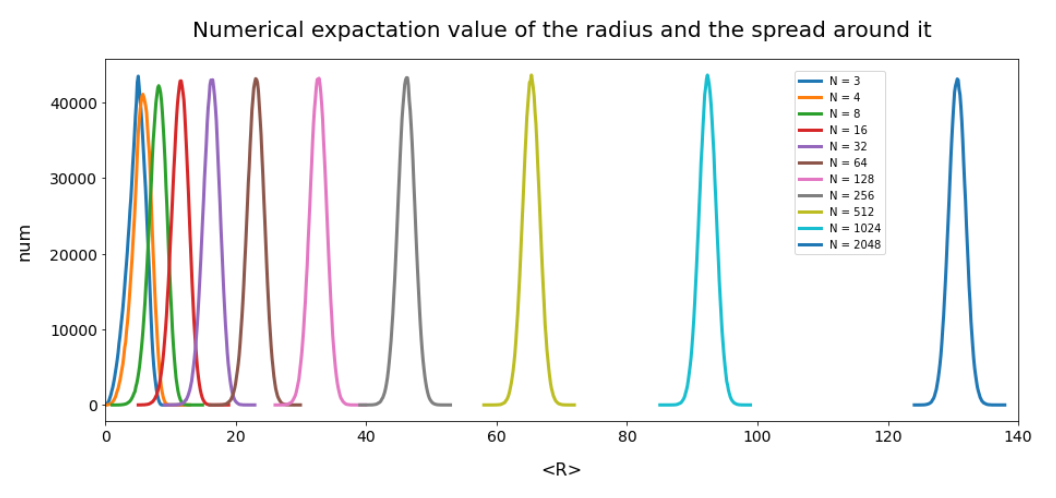Note that one should be careful not to assume more than a point concentration in regions of a multi-dimensional spherical shell. Even within such a shell the created data points may avoid certain regions. I will give some arguments that support this point of view in the next post. But already now it has become very clear that a statistical distribution of vectors with constant probabilities of component values per dimension will not at all fill the latent space homogeneously. # Some better approximations for <R> and the relative standard deviation The above formulas suffer from the fact that $\sqrt{\lt R^{\,2} \gt} \: \gtrsim \: \lt R \gt$ is only a rough approximation which overestimates the real$lt; R > – value, which comes out of numerical simulations. The deviation rises sharply with a small number of dimensions. For a thorough discussion of how good the approximation is see the answer and links at the following discussion at stackexchange
https://stats.stackexchange.com/ questions/ 317095/ expectation-of-square-root-of-sum-of-independent-squared-uniform-random-variable

Below I give you a better approximation to < R >, which should be sufficient for most purposes and N ≥ 3:

$\lt R \gt \: \approx \: = \: b * \sqrt{ 1 /3 * N \,} * \sqrt{{1 \over {1 + {1 \over 4N} }} }$

This approximation can in turn be used to improve the ratio of the standard deviation to < N gt;:

${1 \over {\left< R \right>} } * \sqrt{ \left< \, \left( \, R \, – \lt R \gt \, \right)^{2} \, \right> \,} \: \approx \: \sqrt{ 1 / (5N) \, } * {1 \over {1 + {1 \over 4N} }}$

# Conclusion and outlook on the next post

In this post I have tried to fill a cube around the origin of a multi-dimensional orthogonal coordinate system with statistical points. I have assumed a constant probability density for each component of the respective vectors. A simple mathematical analysis leads to the somewhat surprising conclusion that the resulting data distribution concentrates in regions which are located within a multi-dimensional spherical shell. This shell becomes narrower and narrower with a rising number of dimensions N.

In the next post

Latent spaces – pitfalls of distributing points in multi dimensions – II – missing specific regions

I shall first prove the derived results and given approximations by numerical data. Then I will discuss what kind of regions, that a Neural Network might fill in its latent space, we will miss by such an approach.

# Autoencoders, latent space and the curse of high dimensionality – II – a view on fragments and filaments of the latent space for CelebA images

I continue with experiments regarding the structure which an Autoencoder [AE] builds in its latent space. In the last post of this series

Autoencoders, latent space and the curse of high dimensionality – I

we have trained an AE with images of the CelebA dataset. The Encoder and the Decoder of the AE consist of a series of convolutional layers. Such layers have the ability to extract characteristic patterns out of input (image) data and save related information in their so called feature maps. CelebA images show human heads against varying backgrounds. The AE was obviously able to learn the typical features of human faces, hair-styling, background etc. After a sufficient number of training epochs the AE’s Encoder produces “z-points” (vectors) in the latent space. The latent space is a vector space which has a relatively low number of dimension compared with the number of image pixels. The Decoder of the AE was able to reconstruct images from such z-points which resembled the original closely and with good quality.

We saw, however, that the latent space (or “z-space”) lacks an important property:

The latent space of an Autoencoder does not appear to be densely and uniformly populated by the z-points of the training data.

We saw that his makes the latent space of an Autoencoder almost unusable for creative and generative purposes. The z-points which gave us good reconstructions in the sense of recognizable human faces appeared to be arranged and positioned in a very special way within the latent space. Below I call a CelebA related z-point for which the Decoder produces a reconstruction image with a clearly visible face a “meaningful z-point“.

We could not reconstruct “meaningful” images from randomly chosen z-points in the latent space of an Autoencoder trained on CelebA data. Randomly in the sense of random positions. The Decoder could not re-construct images with recognizable human heads and faces from almost any randomly positioned z-point. We got the impression that many more non-meaningful z-points exist in latent space than meaningful z-points.

We would expect such a behavior if the z-points for our CelebA training samples were arranged in tiny fragments or thin (and curved) filaments inside the multidimensional latent space. Filaments could have the structure of

• multi-dimensional manifolds with almost no extensions in some dimensions
• or almost one-dimensional string-like manifolds.

The latter would basically be described by a (wiggled) thin curve in the latent space. Its extensions in other dimensions would be small.

It was therefore reasonable to assume that meaningful z-points are surrounded by areas from which no reasonable interpretable image with a clear human face can be (re-) constructed. Paths from a “meaningful” z-point would only in a very few distinct directions lead to another meaningful point. As it would be the case if you had to follow a path on a thin curved manifold in a multidimensional vector space.

So, we had some good reasons to speculate that meaningful data points in the latent space may be organized in a fragmented way or that they lie within thin and curved filaments. I gave my readers a link to a scientific study which supported this view. But without detailed data or some visual representations the experiments in my last post only provided indirect indications of such a complex z-point distribution. And if there were filaments we got no clue whether these were one- or multidimensional.

I have to correct this post regarding the basic line of thought: Even if we find that the z-points for CelebA images are arranged in filaments the failure we saw in the first post of this series may not have its direct cause in missing these filaments in latent space by randomly chosen z-points. It could also be that we miss a much larger, coherent region where meaningful points are located. The filaments then would correspond to a correlation of certain features, only, which may not be decisive for the reconstruction of a face. So, the investigation of the existence of filaments is interesting – but the explanation of the AE’s reconstruction failure may require a more thorough analysis. I have done the calculations already, but have not yet found the time to write about them. As soon as the posts are ready I am going to provide a link. See also an added comment at the end of this post.

Do we have a chance to get a more direct evidence about a fragmented or filamental population of the latent space? Yes, I think so. And this is the topic of this post.

However, the analysis is a bit complicated as we have to deal with a multidimensional space. In our case the number of dimensions of the latent space is z_dim = 256. No chance to plot any clusters or filaments directly! However, some other methods will help to reduce the dimensionality of the problem and still get some valid representations of the data point correlations. In the end we will have a very strong evidence for the existence of filaments in the AE’s z-space.

# Methods to work with data distributions in many dimensions

Below I will use several methods to investigate the z-point distribution in the multidimensional latent space:

• An analysis of the variation of the z-point number-density along coordinate axes and vs. radius values.
• An application of t-SNE projections from the standard multidimensional coordinate system onto a 2-dimensional plane.
• PCA analysis and subsequent t-SNE projections of the PCA-transformed z-point distribution and its most important PCA components down to a 2-dim plane. Note that such an approach corresponds to a sequence of projections:
1) Linear projections onto PCA rotated coordinates.
2) A non-linear SNE-projection which scales and represents data point correlations on different scales on a 2-dim plane.
• A direct view on the data distribution projected onto flat planes formed by two selected coordinate axes in the PCA-coordinate system. This will directly reveal whether the data (despite projection effects exhibit filaments and voids on some (small ?) scales.
• A direct view on the data distribution projected onto a flat plane formed by two coordinate axes of the original latent space.

The results of all methods combined strongly support the claim that the latent space is neither populated densely nor uniformly on (small) scales. Instead data points are distributed along certain filamental structures around voids.

# Layer structure of the Autoencoder

Below you find the layer structure of the AE’s Encoder. It got four Conv2D layers. The Decoder has a corresponding reverse structure consisting of Conv2DTranspose layers. The full AE model was constructed with Keras. It was trained on CelebA for 24 epochs with a small step size. The original CelebA images were reduced to a size of 96×96 pixels.

# Number density of z-points vs. coordinate values

Each z-point can be described by a vector, whose components are given by projections onto the 256 coordinate axes. We assume orthogonal axes. Let us first look at the variation of the z-point number density vs. reasonable values for each of the 256 vector-components.

Below I have plotted the number density of z-points vs. coordinate values along all 256 coordinate axes. Each curve shows the variation along one of the 256 axes. The data sampling was done on intervals with a width of 0.25: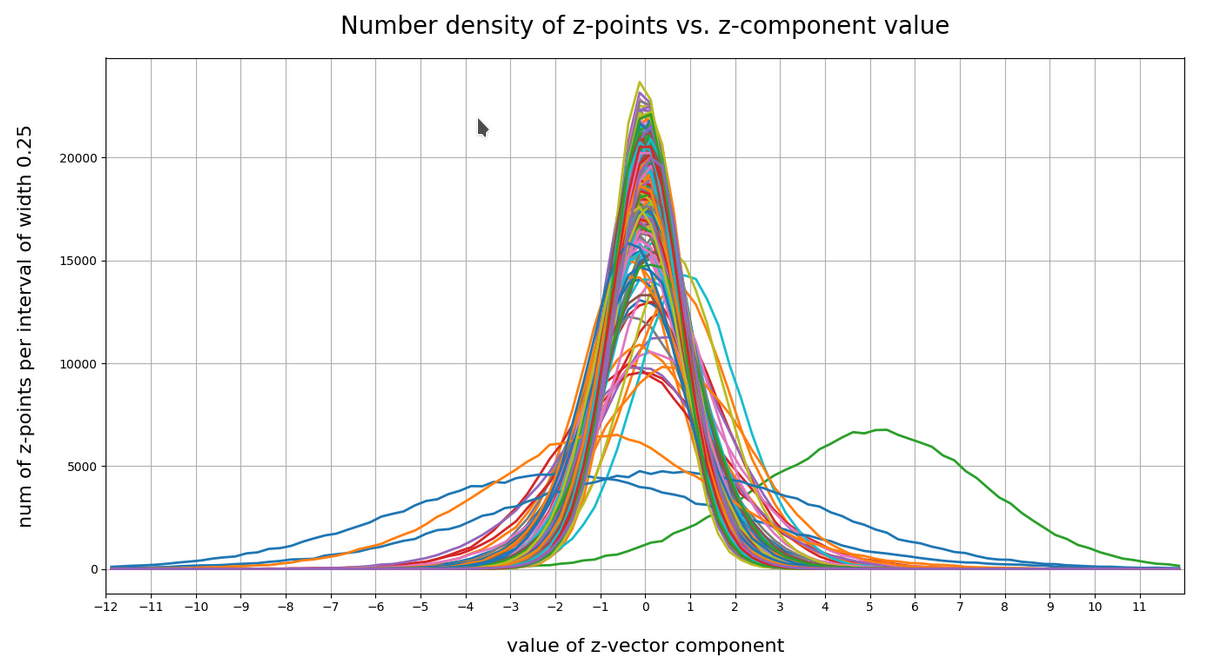Most curves look like typical Gaussians with a peak at the coordinate value 0.0 with a half-width of around 2.

You see, however, that there are some coordinates which dominate the spatial distribution in the latent vector-space. For the following components the number density distribution is relatively broad and peaks at a center different from the origin of the z-space. To pick a few of these coordinate axes:

 52, center:  5.0,  width: 8
61; center;  1.0,  width: 3
73; center:  0.0,  width: 5.5
83; center: -0.5,  width: 5
94; center:  0.0,  width: 4
116; center:  0.0,  width: 4
119; center:  1.0,  width: 3
130; center: -2.0,  width: 9
171; center:  0.7,  width: 5
188; center:  0.75, width: 2.75
200; center:  0.5,  width: 11
221; center: -1.0,  width: 8


The first number is just an index of the vector component and the related coordinate axis. The next plot shows the number density along some these specific coordinate axes:

What have we learned?
For most coordinate axes of the latent space the number density of the z-points peaks at 0.0. We see an approximate Gaussian form of the number density distribution. There are around 5 coordinate directions where the distribution has a peak significantly off the origin (52, 130, 171, 200, 221). Along the corresponding axes the distribution of z-points obviously has an elongated form.

If there were only one such special vector component then we would speak of an elongated, ellipsoidal and almost cigar like distribution with the thickest area at some position along the specific coordinate axis. For a combination of more axes with elongated distributions, each with with a center off the origin, we get instead diagonally oriented multidimensional and elongated shapes.

These findings show again that large regions of the latent space of an AE remain empty. To get an idea just imagine a three dimensional space with all data in x-direction culminating at a coordinate value of 5 with a half-width of lets say 8. In the other directions y and z we have our Gaussian distributions with a total half-width of 1 around the mean value 0. What do we get? A cigar like shape confined around the x-axis and stretching from -3 < x < 13. And the rest of the space: More or less empty. We have obviously found something similar at different angular directions of our multidimensional latent space. As the number of special coordinate directions is limited these findings tell us that a PCA analysis could be helpful. But let us first have a look at the variation of number density with the radius value of the z-points.

# Number density of z-points vs. radius

We define a radius via an Euclidean L2 norm for our 256-dimensional latent space. Afterward we can reduce the visualization of the z-point distribution to a one dimensional problem. We can just plot the variation of the number density of z-points vs. the radius of the z-points.

In the first plot below the sampling of data was done on intervals of 0.5 .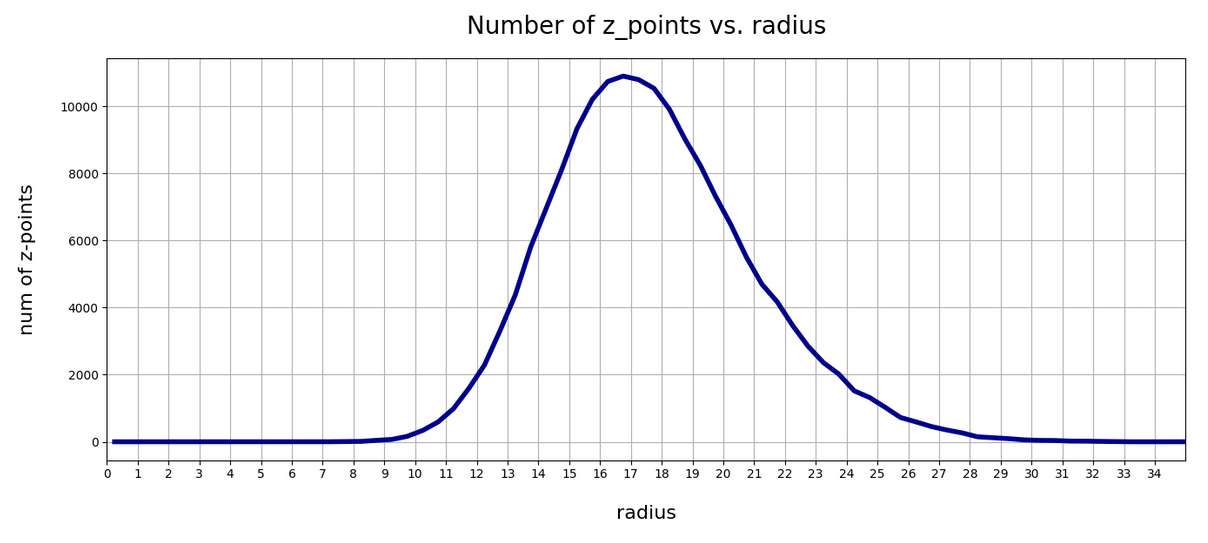The curve does not remain that smooth on smaller sampling intervals. See e.g. for intervals of width 0.05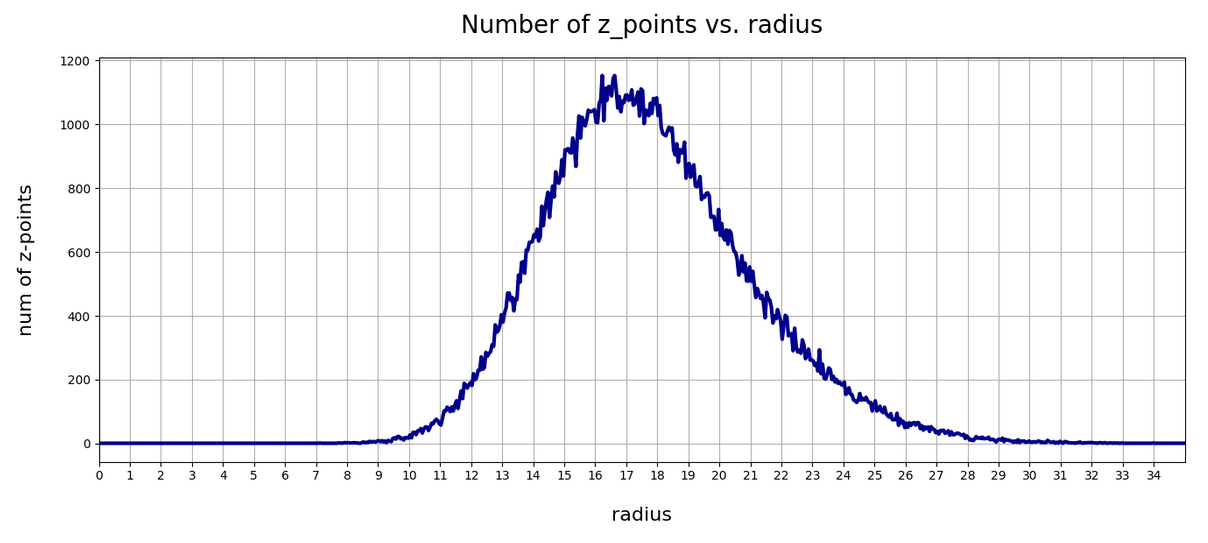Still, we find a pronounced peak at a radius of R=16.5. But do not get misguided: 16 appears to be a big value. But this is mainly due to the high number of dimensions!

How does the peak in the close vicinity of R=16 fit to the above number density data along the coordinate axes? Answer: Very well. If you assume a z-point vector with an average value of 1 per coordinate direction we actually get a radius of exactly R=16!

But what about Gaussian distributions along the coordinate axes? Then we have to look at resulting expectation values. Let us assume that we fill a vector of dimension 256 with numbers for each component picked statistically from a normal distribution with a width of 1. And let us repeat this process many times. Then what will the expectation value for each component be?

A coordinate value contributes with its square to the radius. The math, therefore, requires an evaluation of the integral integral[(x**2)*gaussian(x)] per coordinate. This integral gives us an expectation value for the contribution of each coordinate to the total vector length (on average). The integral indeed has a resulting value of 1.0. From this it follows that the expectation value for the distance according to an Euclidean L2-metric would be avg_radius = sqrt(256) = 16. Nice, isn’t it?

However, due to the fact that not all Gaussians along the coordinate axes peak at zero, we get, of course, some deviations and the flank of the number distribution on the side of larger radius values becomes relatively broad.

What do we learn from this? Regions very close to the origin of the z-space are not densely populated. And above a radius value of 32, we do not find z-points either.

# t-SNE correlation analysis and projections onto a 2-dimensional plane

To get an impression of possible clustering effects in the latent space let us apply a t-SNE analysis. A non-standard parameter set for the sklearn-variant of t-SNE was chosen for the first analysis

tsne2 = TSNE(n_components, early_exaggeration=16, perplexity=10, n_iter=1000)


The first plot shows the result for 20,000 randomly selected z-points corresponding to CelebA images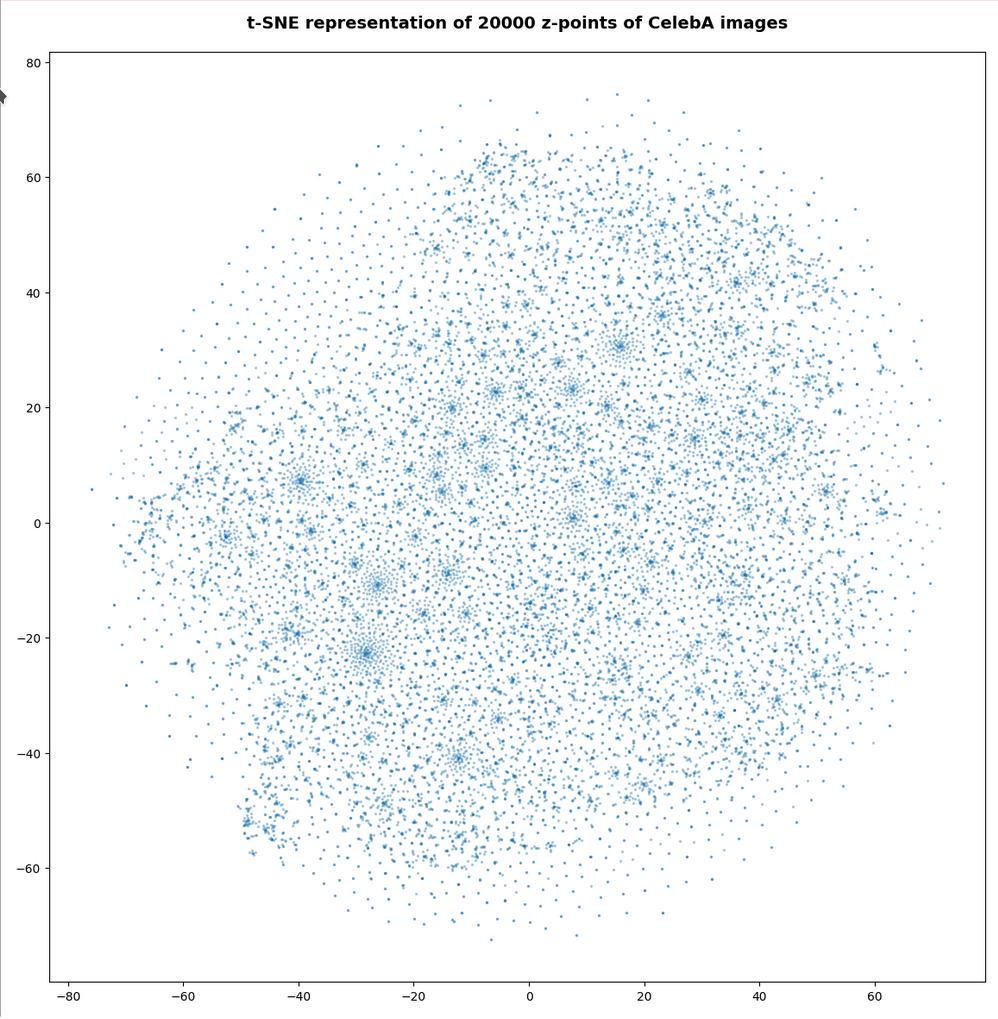Also this plot indicates that the latent space is not populated with uniform density in all regions. Instead we see some fragmentation and clustering. But note that this might happened on different length scales. t-SNE arranges its projections such that correlations on different scales get clearly indicated. So the distances in this plot must not be confused with the real spatial distances in the original latent space. The axes of the t-SNE plot do not reflect any axes of the latent space and the plotted distribution is not the real data point distribution after a linear and orthogonal projection onto a plane. t-SNE works non-linearly.

However, the impression of clustering remains for a growing numbers of z-points. In contrast to the first plot the next plots for 80,000 and 165,000 z-points were calculated with standard t-SNE parameters.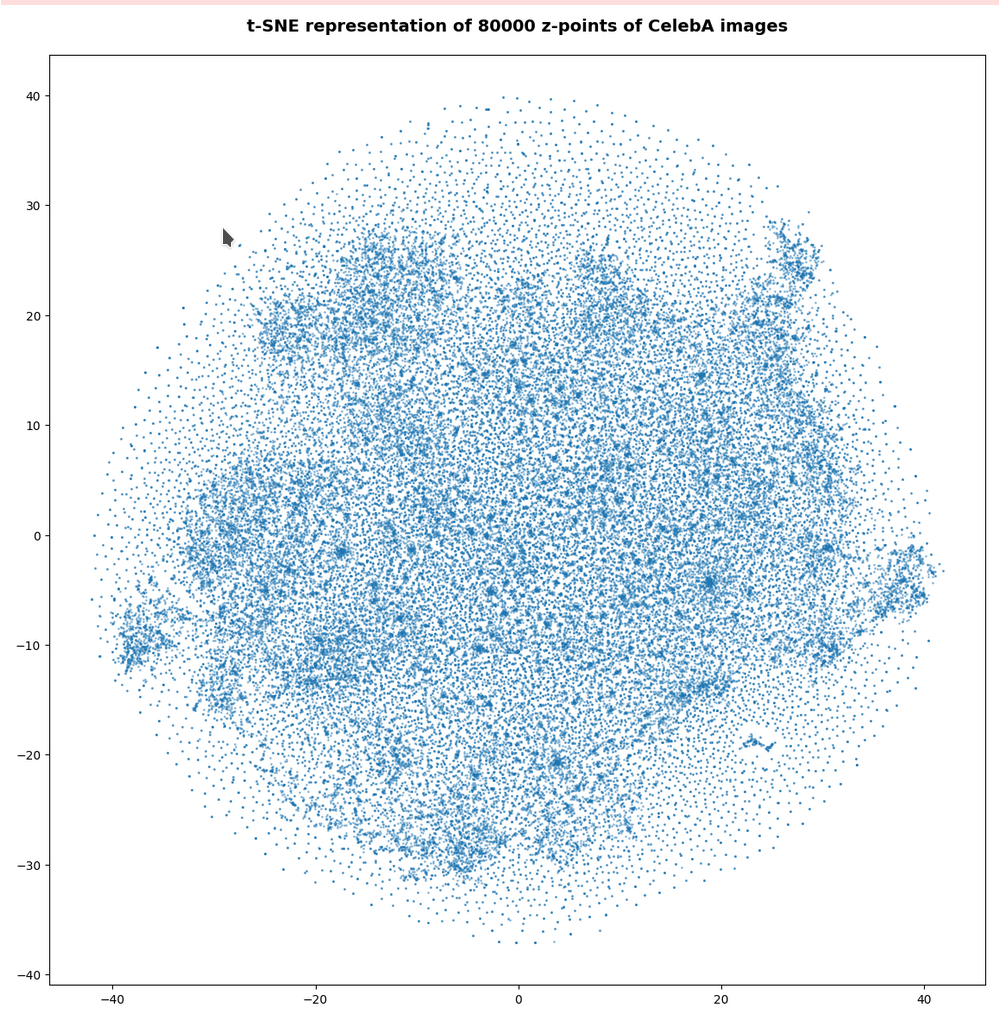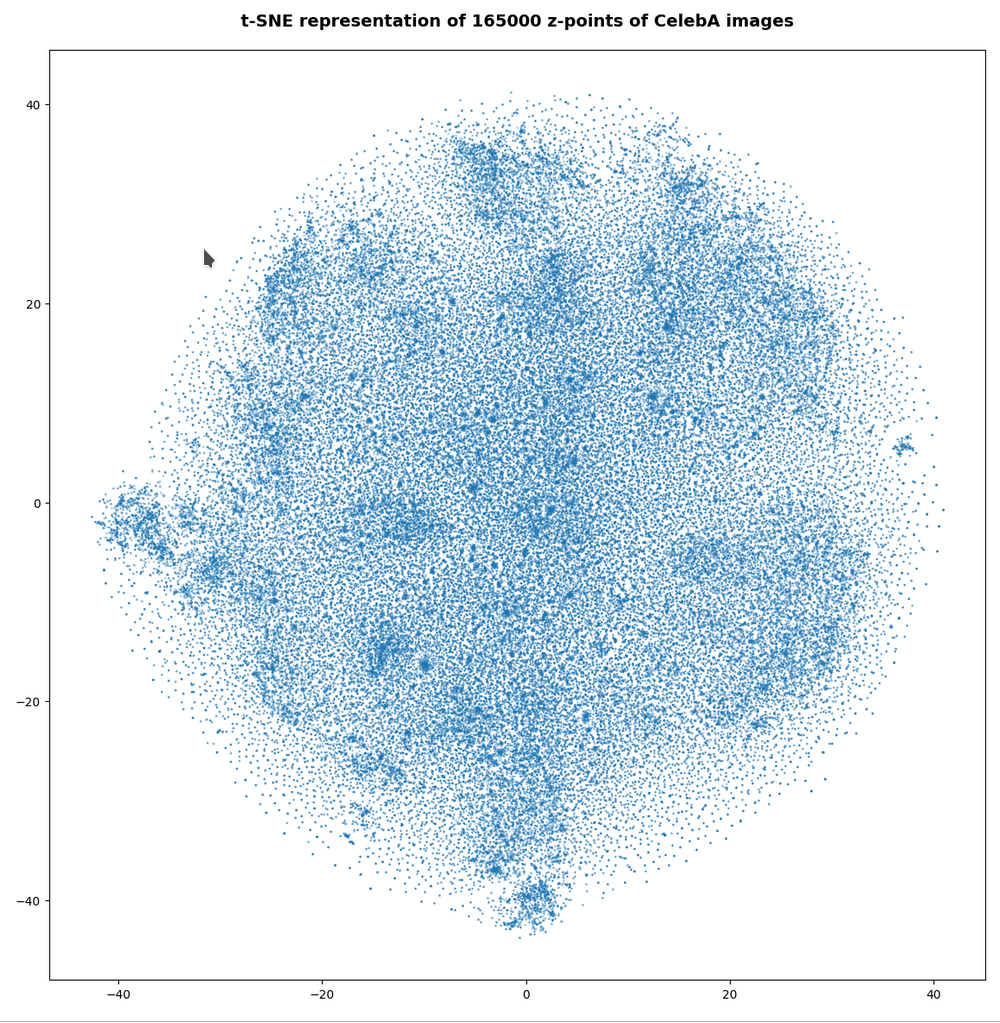We still see gaps everywhere between locally dense centers. At the center the size of the plotted points leads to overlapping. If one could zoom into some of the centers then gaps would again appear on smaller scales (see more plots below).

# PCA analysis and t-SNE-plots of the z-point distribution in the (rotated) PCA coordinate system

The z-point distribution can be analyzed by a PCA algorithm. There is one dominant component and the importance smooths out to an almost constant value after the first 10 components.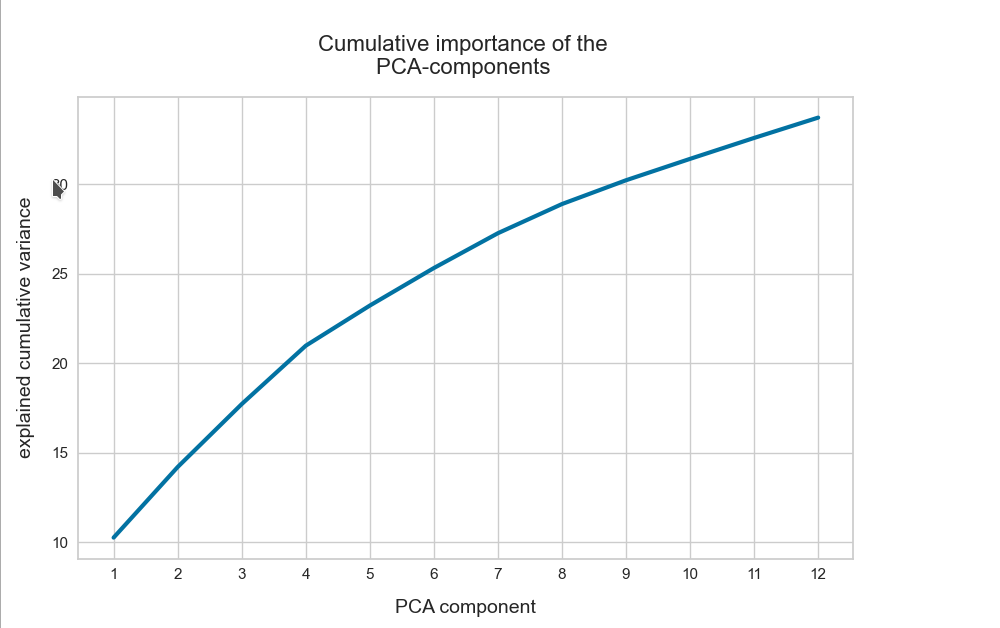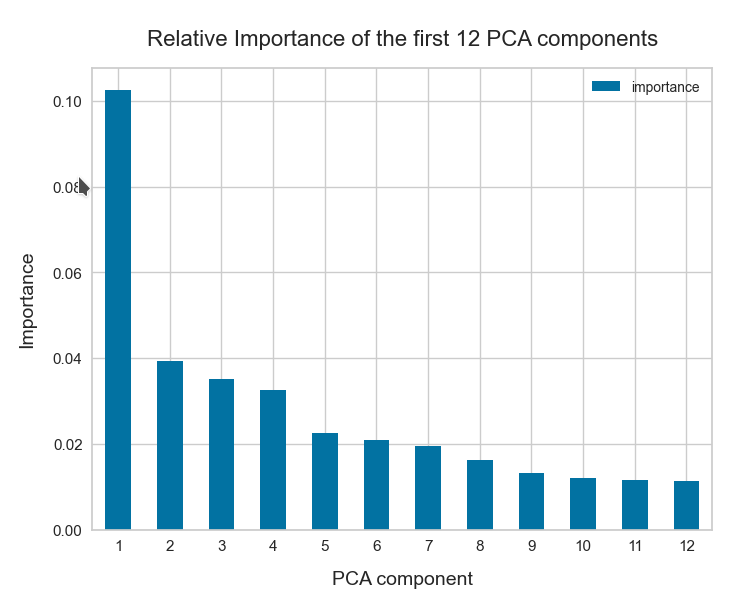This is consistent with the above findings. Most of the coordinates show rather similar Gaussian distributions and thus contribute in almost the same manner.

The PCA-analysis transforms our data to a rotated coordinate system with a its origin at a position such that the transformed z-point distribution gets centered around this new origin. The orthogonal axes of the new PCA-coordinates system show into the direction of the main components.

When the projection of all points onto planes formed by two selected PCA axes do not show a uniform distribution but a fragmented one, then we can safely assume that there really is some fragmentation going on.

## t-SNE after PCA

Below you see t-SNE-plots for a growing number of leading PCA components up to 4. The filamental structure gets a bit smeared out, but it does not really disappear. Especially the elongated empty regions (voids) remain clearly visible.

t-SNE after PCA for the first 2 main components – 80,000 randomly selected z-points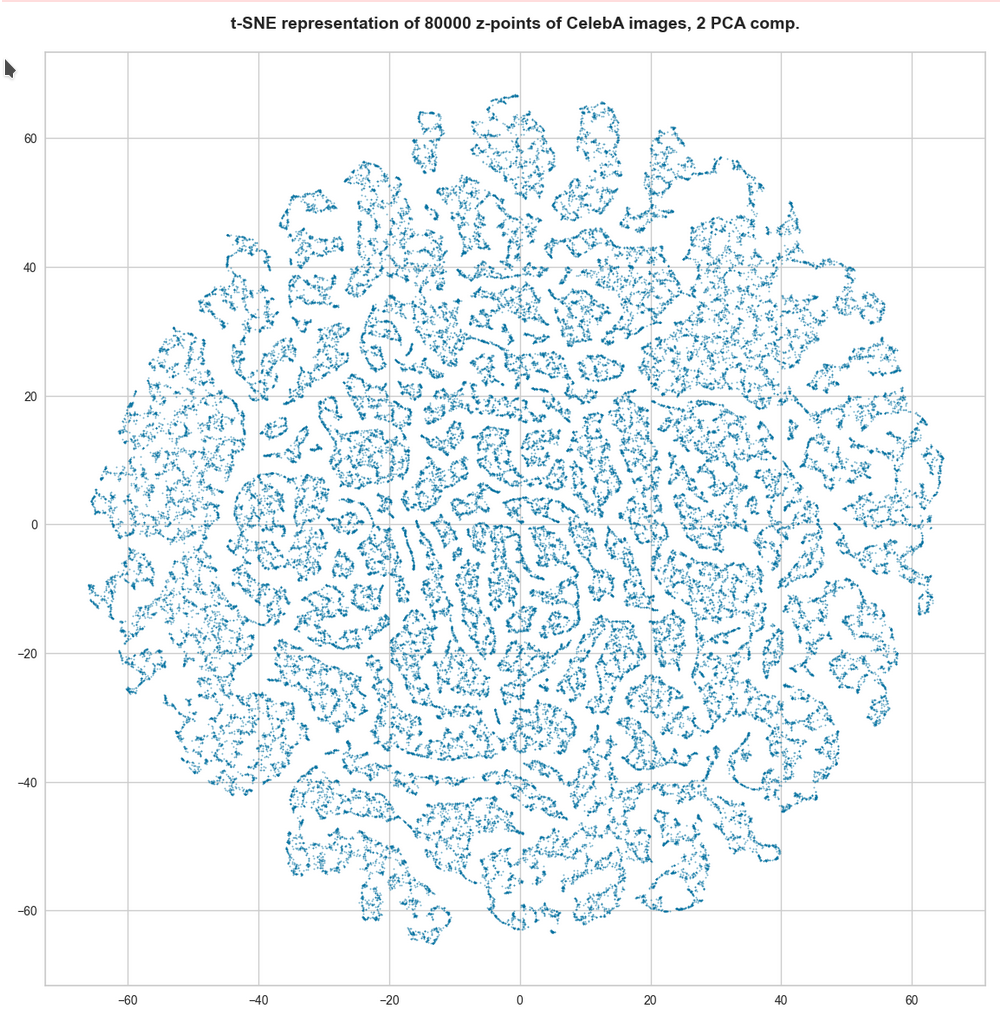t-SNE after PCA for the first 2 main components – 165,000 randomly selected z-points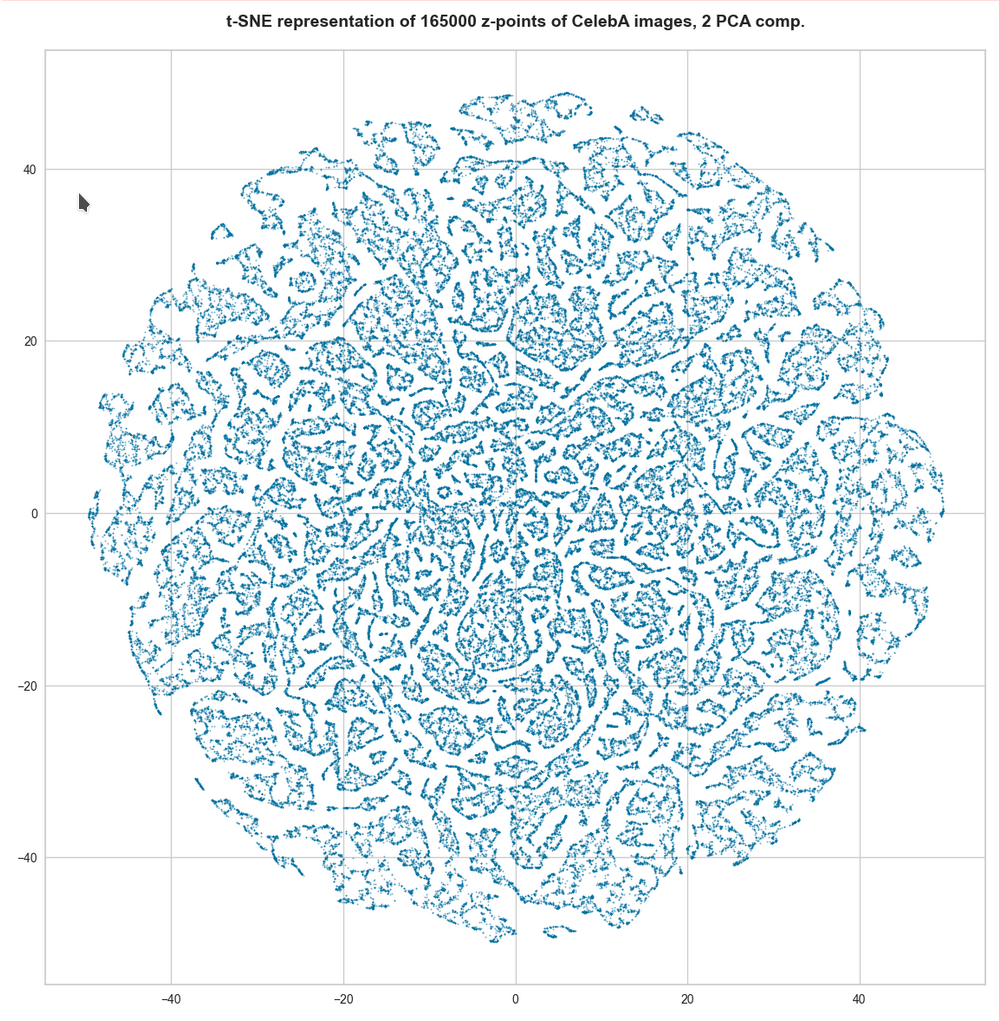t-SNE after PCA for the first 4 main PCA components – 165,000 randomly selected z-points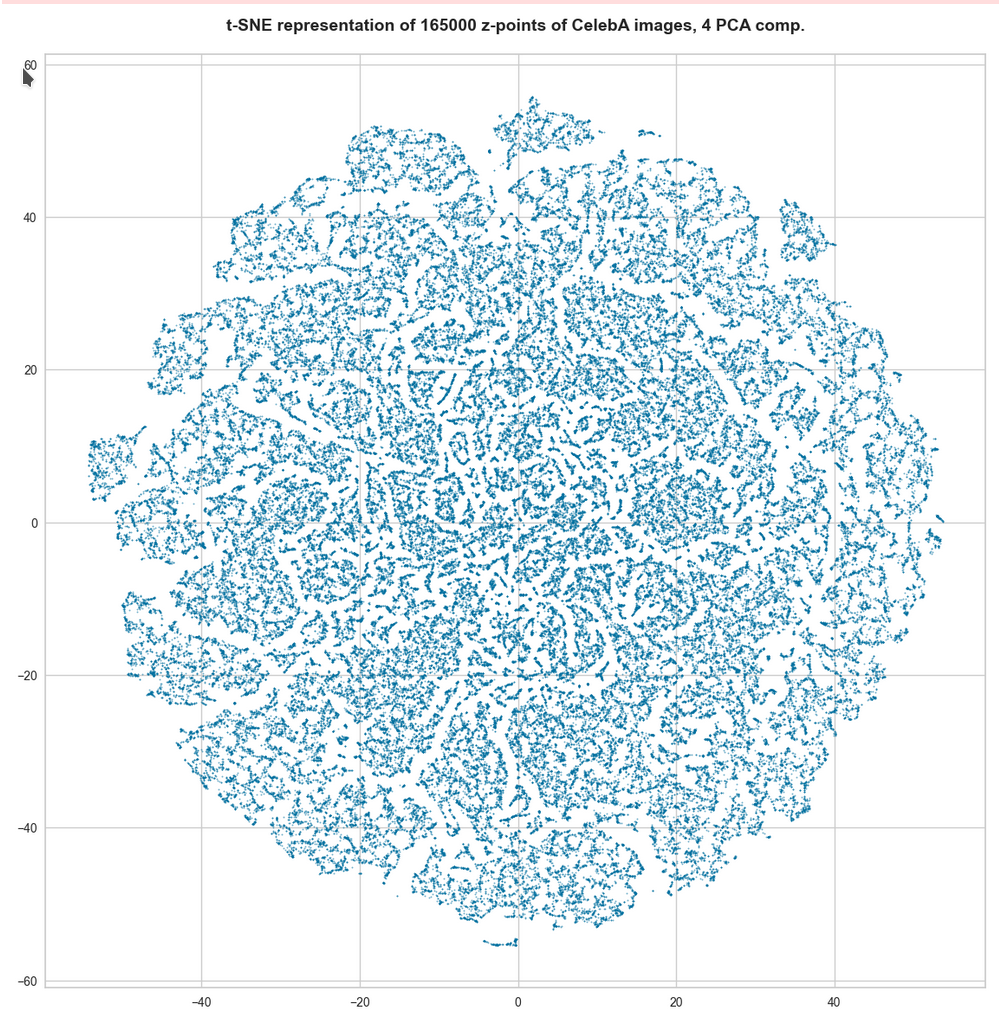For 10 components t-SNE gets a presentation problem and the plots get closer to what we saw when we directly operated on the latent space.But still the 10-dim space does not appear to be uniformly populated. Despite an expected smear out effect due to the non-linear projection the empty ares seem to be at least as many and as extended as the populated areas.

# Direct view on the z-point distribution after PCA in the rotated and centered PCA coordinate system

t-SNE blows correlations up to make them clearly visible. Therefore, we should also answer the following question:

On what scales does the fragmentation really happen ?

For this purpose we can make a scatter plot of the projection of the z-points onto a plane formed by the leading two primary component axes. Let us start with an overview and relatively large limiting values along the two (PCA) axes: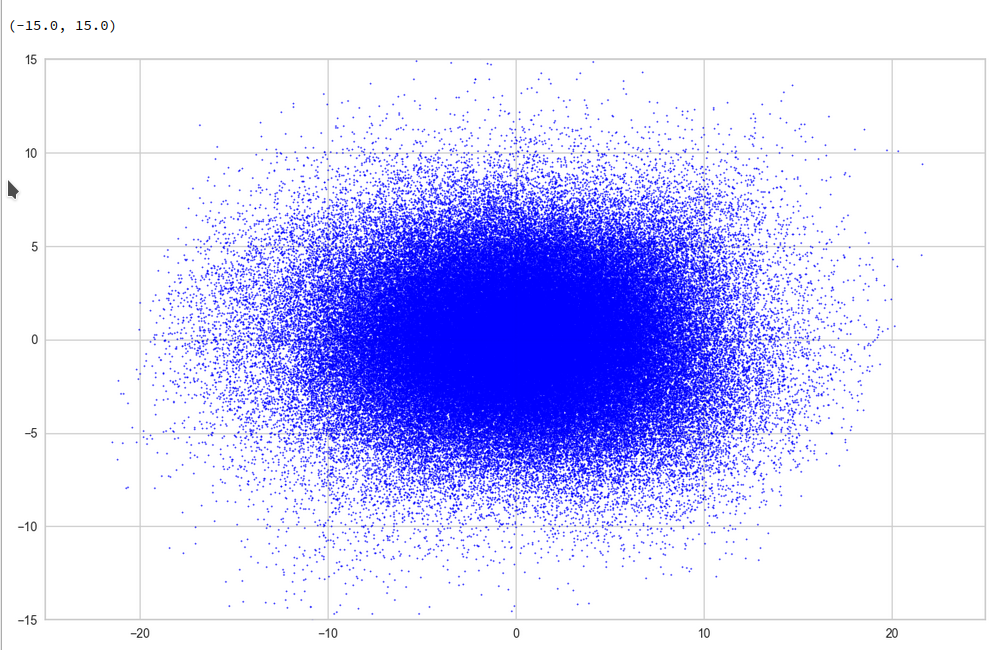Yeah, a PCA transformation obviously has centered the distribution. But now the latent space appears to be filled densely and uniformly around the new origin. Why?

Well, this is only a matter of the visualized length scales. Let us zoom in to a square of side-length 5 at the center: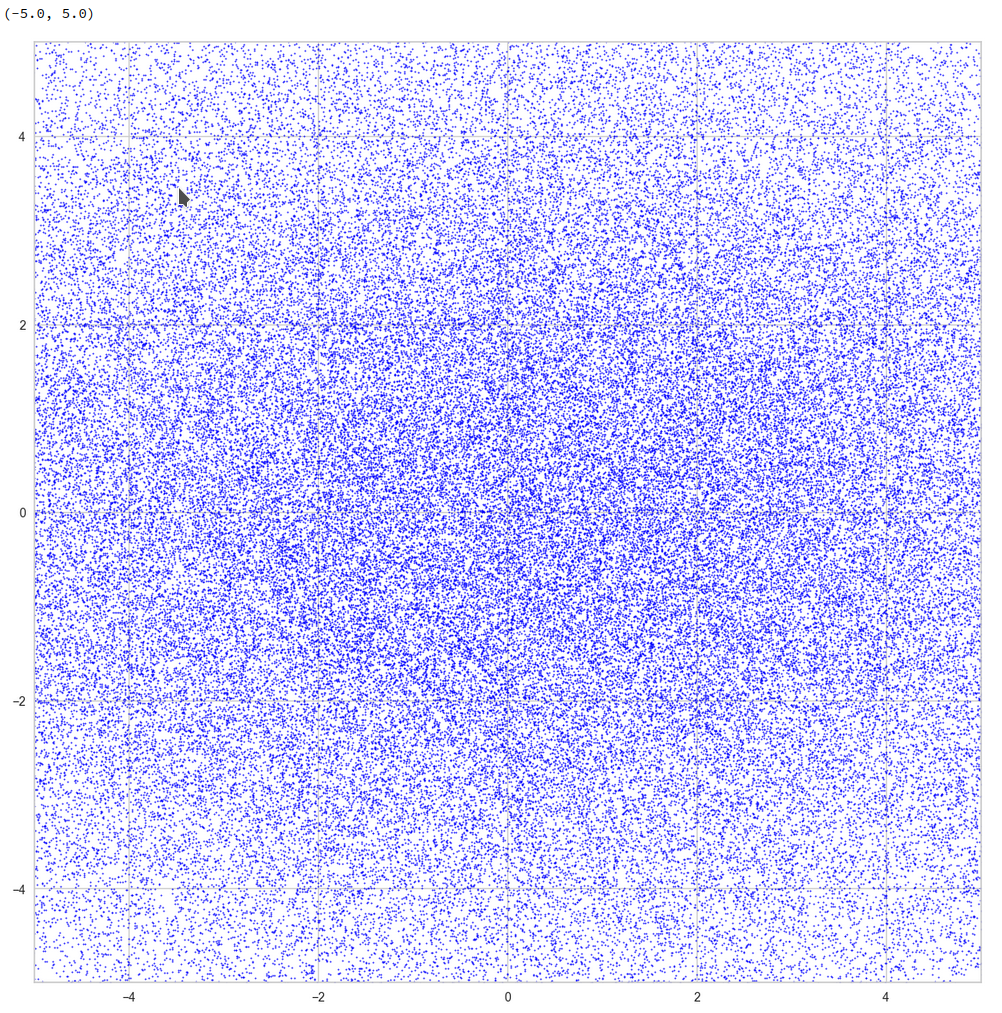Well, not so densely populated as we thought.

And yet a further zoom to smaller length scales: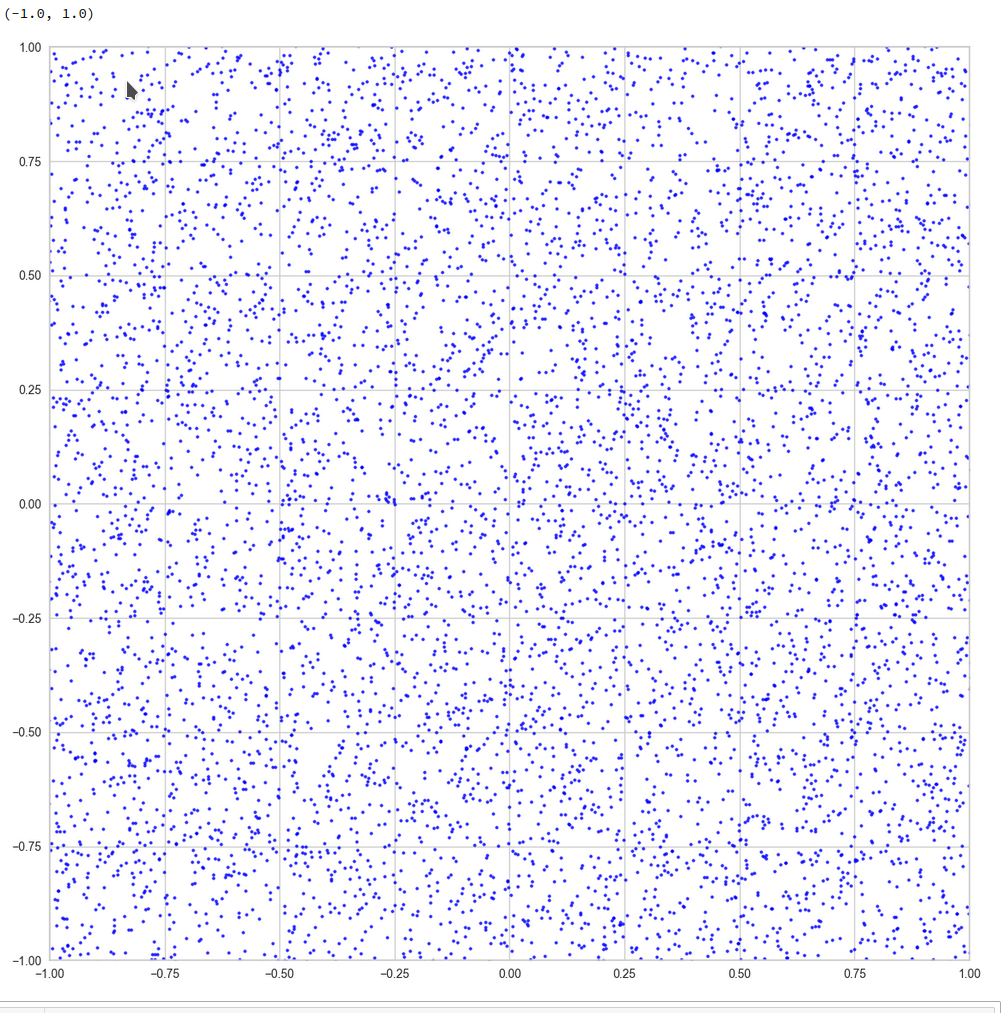And eventually a really small square around the origin of the PCA coordinate system:

z-point distribution at the center of a two-dim plane formed by the coordinate axes of the first 2 primary components
The chosen qsquare has its corners at (-0.25, -0.25), (-0.25, 0.25), (0.25, -0.25), (0.25, 0.25).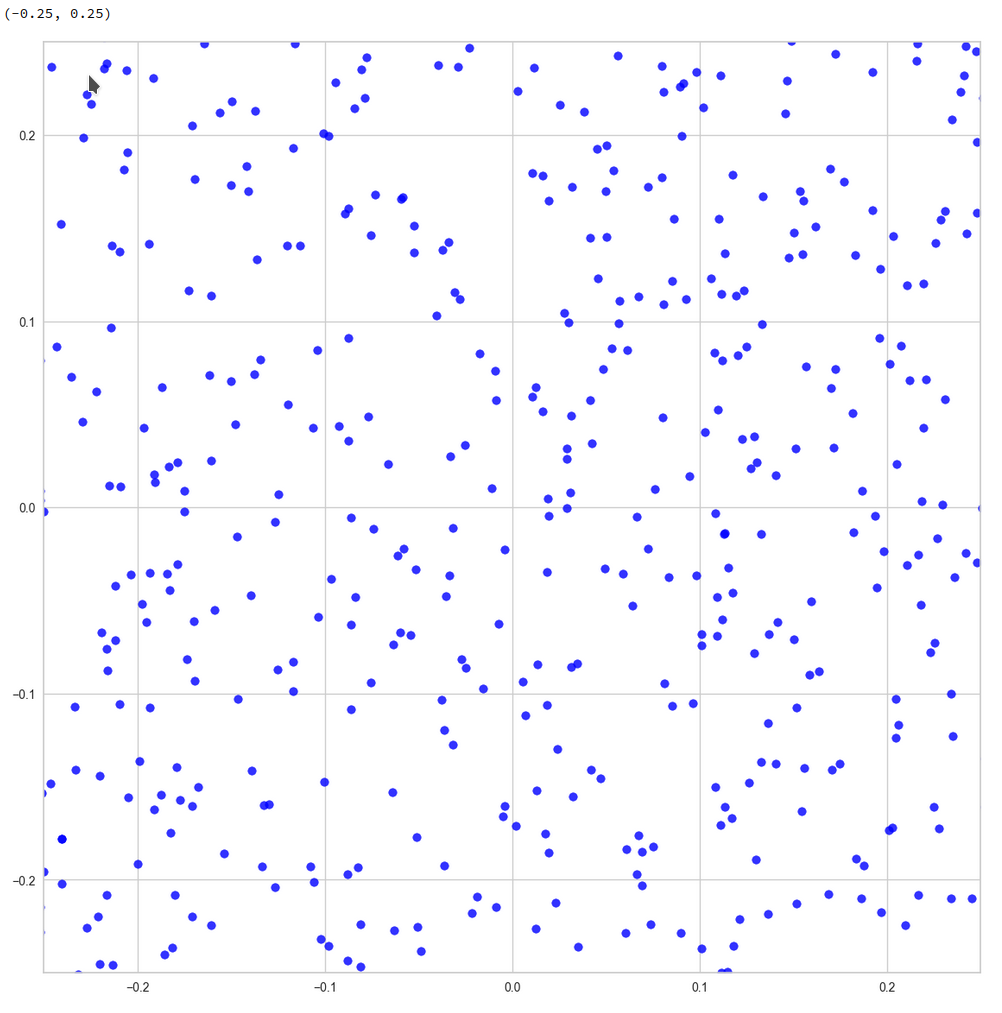Obviously, not a dense and neither a uniform distribution! After a PCA transformation we see the still see how thinly the latent space is populated and that the “meaningful” z-points from the CelebA data lie along curved and narrow lines or curves with some point-like intersections. Between such lines we see extended voids.

Let us see what happens when we look at the 2-dim pane defined by the first and the 18th axes of the PCA coordinate system: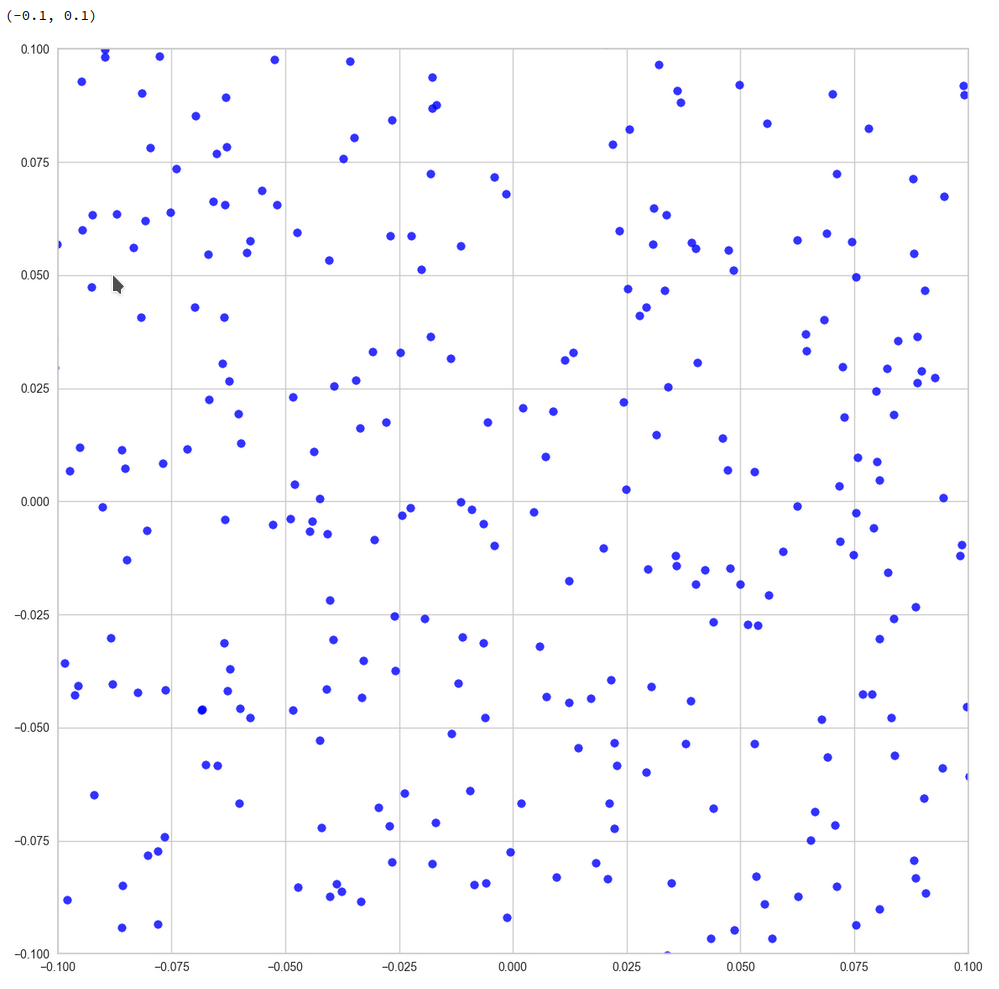Or the distribution resulting for the plane formed by the 8th and the 35th PCA axis: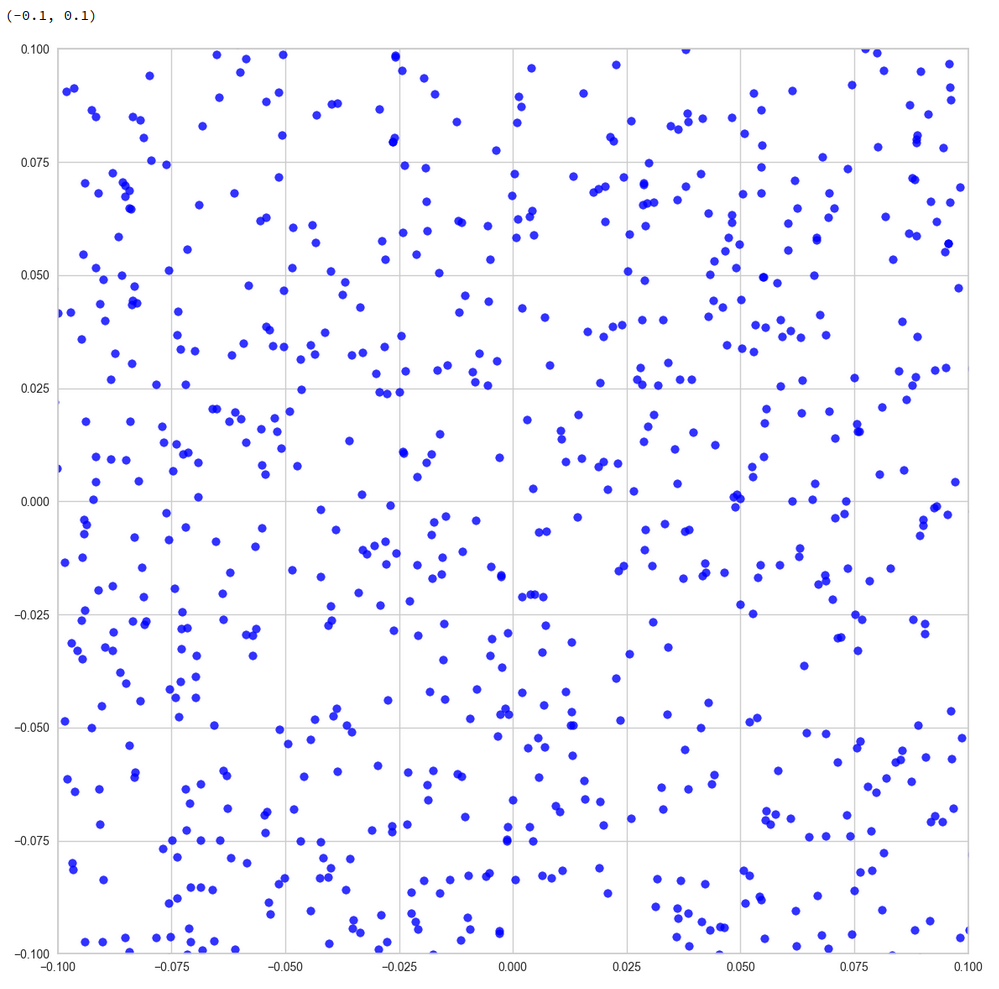We could look at other flat planes, but we do not get rid of he line like structures around void like areas. This is really a strong indication of filamental structures.

Interpretation of the line patterns:
The interesting thing is that we get lines for z-point projections onto multiple planes. What does this tell us about the structure of the filaments? In principle we have the two possibilities already named above: 1) Thin multidimensional manifolds or 2) thin and basically one-dimensional manifolds. If you think a bit about it, you will see that projections of multidimensional manifolds would not give us lines or curves on all projection planes. However curved string- or tube-like manifolds do appear as lines or line segments after a projection onto almost all flat planes. The prerequisite is that the extension of the string in other directions than its main one must really be small. The filament has to have a small diameter in all but one directions.

So, if the filaments really are one-dimensional string-like objects: Should we not see something similar in the original z-space? Let us for example look at the plane formed by axis 52 and axis 221 in the original z-space (without PCA transformation). You remember that these were axes where the distribution got elongated and had centers at -2 and 5, respectively. And indeed:

Again we see lines and voids. And this strengthens our idea about filaments as more or less one-dimensional manifolds.

The “meaningful” z-points for our CelebA data obviously get positioned on long, very thin and basically one-dimensional filaments which surround voids. And the voids are relatively large regarding their area/volume. (Reminds me of the galaxy distribution in simulations of the development of the early universe, by the way.)

Therefore: Whenever you chose a randomly positioned z-point the chance that you end up in an unpopulated region of the z-space or in a void and not on a filament is extremely big.

# Conclusion

We have used a whole set of methods to analyze the z-point distribution of an AE trained on CelebA images. We found the the z-point distribution is dominated by the number density variation along a few coordinate axes. Elongated shapes in certain directions of the latent space are very plausible on larger scales.

We found that the number density distributions along most of the coordinate axes have a thin Gaussian form with a peak at the origin and a half-with of 1. We have no real explanation for this finding. But it may be related to the fact the some dominant features of human faces show Gaussian distributions around a mean value. With Gaussians given we could however explain why the number density vs. radius showed a peak close to R=16.

A PCA analysis finds primary directions in the multidimensional space and transforms the z-point distribution into a corresponding one for orthogonal primary components axes. For logical reason we can safely assume that the corresponding projections of the z-point distribution on the new axes would still reveal existing thin filamental structures. Actually, we found lines surrounding voids independently onto which flat plane we projected the data. This finding indicates thin, elongated and curved but basically one-dimensional filaments (like curved strings or tubes). We could see the same pattern of line-like structure in projections onto flat coordinate planes in the original latent space. The volume of the void areas is obviously much bigger than the volume occupied by the filaments.

Non-linear t-SNE projections onto a 2-dim flat hyperplanes which in addition reproduce and normalize correlations on multiple scales should make things a bit fuzzier, but still show empty regions between denser areas. Our t-SNE projections all showed signs of complex correlation patterns of the z-points with a lot of empty space between curved structures.

The following original conclusion is misleading and by parts wrong:

The experiments all in all indicate that z-points of the training data, for which we get good reconstructions, lie within thin filaments on characteristic small length scales. The areas/volumes of the voids between the filaments instead are relatively big. This explains why chances that randomly chosen points in the z-space falls into a void are very high.
The results of the last post are consistent with the interpretation that z-points in the voids do not lead to reconstructions by the Decoder which exhibit standard objects of the training images. in the case of CelebA such z-points do not produce images with clear face or head like patterns. Face like features obviously correspond to very special correlations of z-point coordinates in the latent space. These correlations correspond to thin manifolds consuming only a tiny fraction of the z-space with a volume close zero.

Due to a new analysis I would like to replace my original statemets with a question:

Do our findings of the existence of filaments and large surrounding voids really explain the results of the first post that randomly chosen z-points miss areas in the latent space which allow for a reconstruction of “faces”?

I am going to answer this question in another better prepared post series, soon. To make you a bit curious I leave you with the fact that the following picture shows a face reconstructed by an AE from a randomly selected point in the latent space – with some simple conditions applied: